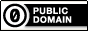## Recommended Posts

I am making a program that will count the number of times different numbers are generated with autoit's random command. When I click start I want the program to begin generating numbers randomly and then counting which number is generated until I hit stop.

```#include <ButtonConstants.au3>
#include <EditConstants.au3>
#include <GUIConstantsEx.au3>
#include <StaticConstants.au3>
#include <WindowsConstants.au3>

Global \$Speed = 100
Global \$Number1 = 0, \$Number2 = 0, \$Number3 = 0, \$Number4 = 0, \$Number5 = 0, \$Number6 = 0, \$Number7 = 0, \$Number7 = 0, \$Number8 = 0, \$Number9 = 0, \$Number10 = 0
Global \$Start = "Start"

#Region ### START Koda GUI section ### Form=
\$Form1 = GUICreate("Form1", 157, 438, 336, 218)
\$Label1 = GUICtrlCreateLabel("1", 24, 176, 10, 17)
\$Label2 = GUICtrlCreateLabel("2", 24, 200, 10, 17)
\$Label3 = GUICtrlCreateLabel("3", 24, 224, 10, 17)
\$Label4 = GUICtrlCreateLabel("4", 24, 248, 10, 17)
\$Label5 = GUICtrlCreateLabel("5", 24, 272, 10, 17)
\$Label6 = GUICtrlCreateLabel("6", 24, 296, 10, 17)
\$Label7 = GUICtrlCreateLabel("7", 24, 320, 10, 17)
\$Label8 = GUICtrlCreateLabel("8", 24, 344, 10, 17)
\$Label9 = GUICtrlCreateLabel("9", 24, 368, 10, 17)
\$Label10 = GUICtrlCreateLabel("10", 24, 392, 16, 17)
\$SpeedLabel = GUICtrlCreateLabel("Speed", 32, 120, 35, 17)
\$Speed = GUICtrlCreateInput(\$Speed, 24, 136, 49, 21)
\$Label11 = GUICtrlCreateLabel(\$Number1, 64, 176, 10, 17)
\$Label12 = GUICtrlCreateLabel(\$Number2, 64, 200, 10, 17)
\$Label13 = GUICtrlCreateLabel(\$Number3, 64, 224, 10, 17)
\$Label14 = GUICtrlCreateLabel(\$Number4, 64, 248, 10, 17)
\$Label15 = GUICtrlCreateLabel(\$Number5, 64, 272, 10, 17)
\$Label16 = GUICtrlCreateLabel(\$Number6, 64, 296, 10, 17)
\$Label17 = GUICtrlCreateLabel(\$Number7, 64, 320, 10, 17)
\$Label18 = GUICtrlCreateLabel(\$Number8, 64, 344, 10, 17)
\$Label19 = GUICtrlCreateLabel(\$Number9, 64, 368, 10, 17)
\$Label20 = GUICtrlCreateLabel(\$Number10, 64, 392, 10, 17)
\$ButtonStart = GUICtrlCreateButton(\$Start, 24, 48, 89, 25)
\$Label21 = GUICtrlCreateLabel("Random Number Count", 16, 16, 115, 17)
\$ButtonResetCount = GUICtrlCreateButton("Reset Count", 24, 80, 89, 25)
GUISetState(@SW_SHOW)
#EndRegion ### END Koda GUI section ###

Do
\$nMsg = GUIGetMsg()
Switch \$nMsg
Case \$GUI_EVENT_CLOSE
Exit 0
Case \$nMsg = \$ButtonStart
StartRandom()
Case \$ButtonResetCount
ResetCount()

EndSwitch
Until \$nMsg = \$GUI_EVENT_CLOSE

Func StartRandom()
If \$Start == "Start" Then
\$Start = "Stop"
Else
\$Start = "Start"
EndIf
;For \$i = 1 To \$Number
While 1
\$RNumber = Random(1,10,1);Random Number
For \$i = 1 To 10
If \$RNumber == 1 Then
\$Number1 += 1
EndIf
If \$RNumber == 2 Then
\$Number2 += 1
EndIf
If \$RNumber == 3 Then
\$Number3 += 1
EndIf
If \$RNumber == 4 Then
\$Number4 += 1
EndIf
If \$RNumber == 5 Then
\$Number5 += 1
EndIf
If \$RNumber == 6 Then
\$Number6 += 1
EndIf
If \$RNumber == 7 Then
\$Number7 += 1
EndIf
If \$RNumber == 8 Then
\$Number8 += 1
EndIf
If \$RNumber == 9 Then
\$Number9 += 1
EndIf
If \$RNumber == 10 Then
\$Number10 += 1
EndIf
Sleep(\$Speed)
Next
WEnd
EndFunc

Func ResetCount()
\$Number1 = 0
\$Number2 = 0
\$Number3 = 0
\$Number4 = 0
\$Number5 = 0
\$Number6 = 0
\$Number7 = 0
\$Number7 = 0
\$Number8 = 0
\$Number9 = 0
\$Number10 = 0
EndFunc```

With the above code I cannot even close the gui even before I try to click start. Can someone with a little more experience with GUI's in Autoit point me to what I am missing?

Get Scite to add a popup when you use a 3rd party UDF -> http://www.autoitscript.com/autoit3/scite/docs/SciTE4AutoIt3/user-calltip-manager.html

##### Share on other sites

computergroove,

Too many loops - you were getting stuck inside the _StartRandom function - and you need to learn about arrays.Try this:

```#include <GUIConstantsEx.au3>

Global \$aLabel, \$bStart = False

\$hGUI = GUICreate("Form1", 157, 438, 336, 218)
GUICtrlCreateLabel("Random Number Count", 16, 16, 115, 17)
For \$i = 1 To 10
GUICtrlCreateLabel("1", 24, 152 + (24 * \$i), 10, 17)
\$aLabel[\$i] = GUICtrlCreateLabel(0, 64, 152 + (24 * \$i), 30, 17)
Next
GUICtrlCreateLabel("Speed", 32, 120, 35, 17)
\$cSpeed = GUICtrlCreateInput(100, 24, 136, 49, 21)
\$cStart = GUICtrlCreateButton("Start", 24, 48, 89, 25)

\$ButtonResetCount = GUICtrlCreateButton("Reset Count", 24, 80, 89, 25)
GUISetState(@SW_SHOW)

\$nTimeStamp = TimerInit()

While 1
Switch GUIGetMsg()
Case \$GUI_EVENT_CLOSE
Exit
Case \$cStart
Case "Start"
GUICtrlSetData(\$cStart, "Stop")
\$bStart = True
Case Else
GUICtrlSetData(\$cStart, "Start")
\$bStart = False
EndSwitch
Case \$ButtonResetCount
ResetCount()
EndSwitch

If \$bStart Then
\$iRandom = Random(1, 10, 1)
\$nTimeStamp = TimerInit()
EndIf
EndIf

WEnd

Func ResetCount()
For \$i = 1 To 10
GUICtrlSetData(\$aLabel[\$i], 0)
Next
\$bStart = False
EndFunc   ;==>ResetCount```
Both the code and the result seem OK to me.M23Any of my own code posted anywhere on the forum is available for use by others without any restriction of any kind

Open spoiler to see my UDFs:

Spoiler

ArrayMultiColSort ---- Sort arrays on multiple columns
ChooseFileFolder ---- Single and multiple selections from specified path treeview listing
Date_Time_Convert -- Easily convert date/time formats, including the language used
ExtMsgBox --------- A highly customisable replacement for MsgBox
GUIExtender -------- Extend and retract multiple sections within a GUI
GUIFrame ---------- Subdivide GUIs into many adjustable frames
GUIListViewEx ------- Insert, delete, move, drag, sort, edit and colour ListView items
GUITreeViewEx ------ Check/clear parent and child checkboxes in a TreeView
Marquee ----------- Scrolling tickertape GUIs
NoFocusLines ------- Remove the dotted focus lines from buttons, sliders, radios and checkboxes
Notify ------------- Small notifications on the edge of the display
Scrollbars ----------Automatically sized scrollbars with a single command
StringSize ---------- Automatically size controls to fit text
Toast -------------- Small GUIs which pop out of the notification area

##### Share on other sites

change line 8 to

`GUICtrlCreateLabel(\$i, 24, 152 + (24 * \$i), 10, 17)`

App: Au3toCmd              UDF: _SingleScript()

## Create an account

Register a new account

• ### Recently Browsing   0 members

×

• Wiki

• Back

• #### Beta

• Git
• FAQ
• Our Picks
×
• Create New...